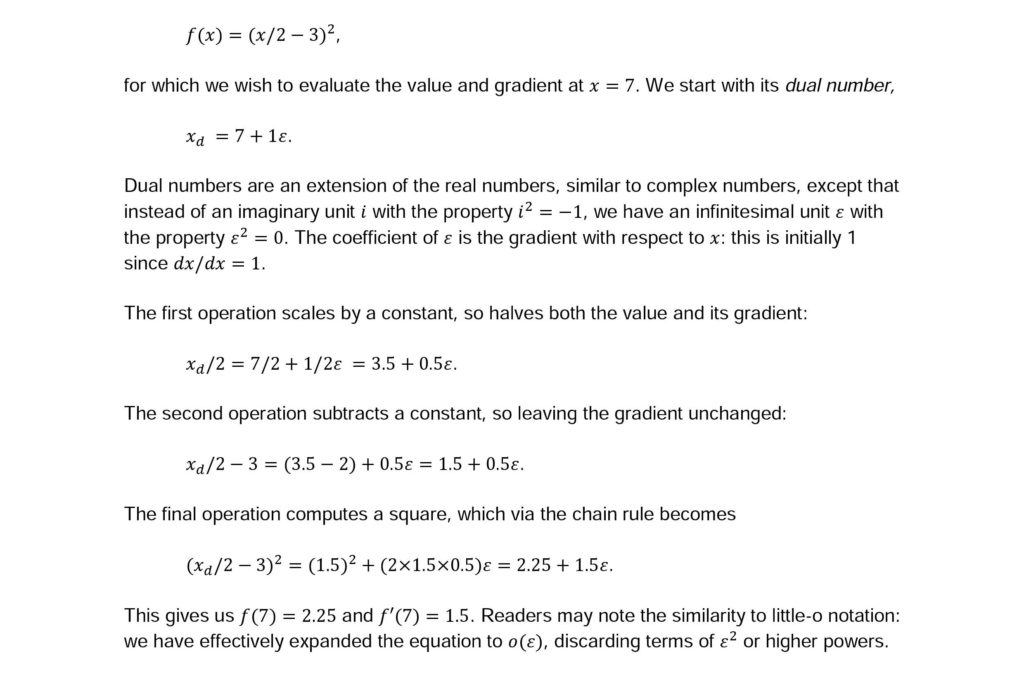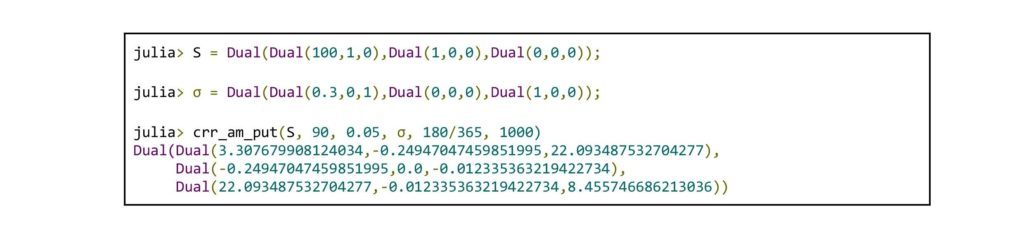# Automatic Differentiation for the Greeks

## Dr. Simon Byrne and Dr. Andrew Greenwell on fast and accurate price sensitivities using JuliaThe sensitivities of the value of an option to the model parameters, a.k.a. “the Greeks,” are crucial to understanding the risk of an option position, as well as tasks such as model calibration.

Outside a few simple cases such as the Black–Scholes model, the Greeks are typically computed using finite-difference approximation. Though often fairly effective, it is rather crude and can become numerically unstable without careful tuning of the difference parameter (it needs to be small enough to reduce discretization error, yet large enough to avoid subtractive cancellation issues), especially for higher-order Greeks. Furthermore, it incurs significant overhead, requiring multiple runs of the program under the same settings and random seeds (at least two for first order, and three or four for second order).

Automatic differentiation (also known as algorithmic differentiation (AD)) is a powerful method for computing gradients and higher-order derivatives of numerical programs, which are both numerically exact, yet incur very little computational overhead. AD has already been explored previously in the quantitative finance literature (see  for a review), but its adoption has been hampered by the difficulty of its use. We showcase the use of AD using the Julia language , a dynamic language whose features make the use of AD both straightforward and highly performant.

## What is Automatic Differentiation?

Before explaining what it is, it is important to emphasize what AD isn’t:

• it isn’t symbolic differentiation, e.g. deriving expressions by hand or via a computer algebra system such as Mathematica;
• it isn’t numerical differentiation, e.g. finite differencing.

Instead, AD uses exactly the same programmatic logic as dictated by the code, except instead of computing just the numerical values of each expression, it propagates along the gradient information via standard properties such as the chain rule. Consider the following example:Though simple mathematically, the point is that the whole process was numeric, and followed the same logic as the original expression: at no point did we need to manipulate the expression symbolically. All these operations can be handled automatically by a computer, as long as it knows how to propagate the gradients. Moreover, it can work directly with the usual control-flow statements of computer programs (if blocks, for loops, etc.).

## Julia: New Adventures in High Performance

Julia is modern, high-performance programming language aimed at numerical computing. In particular, it has several features which make it appealing for use with AD:

• Function overloading via multiple dispatch. We can easily define how the gradients operate on each argument of each function and operator simply by overloading methods. Multiple dispatch makes this easy, safe, and efficient.
• Efficient user-defined types. We can create a Dual type, which is as efficient as built-in types (floats, complex numbers, etc.); indeed, most of Julia itself is written in Julia, including all “primitive” types like integers and floating-point numbers and operations.
• Support for generic programming. We can reuse exactly the same code with different data types. Essentially all of Julia’s standard library is designed to be generic, meaning that if you implement a new number type, you can already use it everywhere.
• High performance. Using tools such as type inference and just-in-time (JIT) compilation, Julia’s performance can be similar to fast static languages like C, C++, and Fortran – you no longer have to pay the “ease-of-use tax” in the form of slow code.

These points explain why AD has not yet been widely used in other languages: C doesn’t support generic functions, and user-defined objects in Java are slow compared with built-in primitives. There are AD libraries in C++, but they require extensive use of template metaprogramming, which has limited their popularity – it’s easier to find an analytic derivative than to work with C++ templates. In Julia you can finally use AD to get derivatives for free without arguing with a compiler.

## AD in Julia: A Binomial Tree

Our first example is a fairly simple implementation of a Cox–Ross–Rubinstein tree for pricing an American put in Julia.

The language is similar to standard dynamic languages such as Python or Matlab – we don’t need to use type annotations. When calling this function with specific argument values, Julia will infer appropriate types based on the actual types of the arguments passed to this function.

Let’s call this function from the interactive Julia prompt:This runs fast: it took 9 ms for a 1000-step tree. For reference, the same example on the same hardware using the C++ library, QuantLib, takes 30 ms – about 3× slower than Julia.

A dual-number implementation is available as part of the ForwardDiff.jl package by Revels et al. . We can load this and compute the delta of the option in conjunction with its value:All we’ve changed was to make the spot price argument to a Dual object instead of a number, and we automatically get back another Dual object, containing both the value and the corresponding gradient. Moreover, this only took 10 ms (i.e., just 10 percent overhead compared with computing just the option value). A finite-difference approach would require at least two complete valuation runs (i.e., 100 percent overhead). Computing with Dual numbers in Julia has similar performance to using complex numbers – which is just as fast as it is in C/C++.

To compute multiple Greeks, we could repeat this multiple times, but the Dual type actually allows multiple partial derivatives to be used:The returned Dual object contains option value, delta, and vega, respectively. This case took 11 ms (20 percent overhead) vs. 200 percent minimum overhead required for finite differencing.

## Hyperduals

The same process can be extended to obtain higher-order derivatives, leading to the notion of a hyperdual number. However, rather than implement this as a whole new number type, we can obtain the same result by simply nesting Dual objects:This returns the value of the option, two first-order Greeks (delta, vega) and three second-order Greeks (gamma, vanna, and vomma). This took 25 ms (170 percent overhead vs. 500 percent for finite differencing).

You may have noticed something odd about these numbers: the gamma term is exactly zero. This turns out to be a property of the binomial model: as S only ever occurs as a linear term in the code, delta is a discontinuous stepwise function, so gamma (where it is defined) is zero (see Figure 1a & 1b). Finite differencing, by comparison, performs terribly in this case, with wildly inaccurate values, varying unpredictably with the choice of step size (see Figure 2).

Figures 1a & 1b: Plots of delta as a function of S from the CRR model. Note the discontinuities at the finer scale.

Figure 2: The estimated values of gamma by finite differencing, for different values of the difference parameter ε. Below 10−5 the error increases exponentially.

A possible solution in this case is to use a likelihood ratio (LR) method [4, section 7.3]. Although this approach is usually applied in the context of Monte Carlo models, it can also be used with deterministic approximations. Unfortunately this can’t be automated easily, since it requires knowledge of the underlying continuous model which the tree is approximating. However, once the LR delta is computed, we can use AD to compute the LR-PW gamma [4, example 7.3.7].

## A Monte Carlo Example

Exactly the same approach can be used with Monte Carlo models. An additional benefit here is that you don’t need to worry about saving and storing random seeds between runs, as is necessary for finite differencing. This is equivalent to computing the pathwise derivatives, but without the need to perform any symbolic manipulation. This is particularly convenient for least-squares Monte Carlo methods (see Example 2), where the derivatives of regression term can be quite fiddly.

The fourth-line call to the typeof function is perhaps the only one that would be out of place in a standard implementation. The purpose of this is to determine the type of result of the computation in the following loop, so that an appropriate array will be allocated. We could hard code a type like Float64, but then we could only use this code for Float64 computations – not Float32 or BigFloat – and for our current application most importantly, not Dual numbers. This small bit of generic programming is necessary to make the code work for different input types.

We can compute the value using 10,000 paths of 1,000 steps:The generic array allocation code allows the routine to work with Dual arguments:The overhead in this case is slightly higher – about 134 percent. Using Dual numbers with linear algebra expressions requires Julia to fall back on generic matrix routines, which are slower than heavily optimized BLAS/LAPACK routines for standard floating-point numbers. Nevertheless, this is still faster (and easier) than finite differencing, and there are further optimizations to linear algebra with dual numbers that can be implemented in the future.

## The Great Beyond

We have really only scratched the surface of the power of automatic differentiation in Julia. The ForwardDiff.jl package  includes a variety of convenience wrappers to compute gradients and Hessians without needing to manually create Dual objects, which is useful for high-dimensional problems, such as multiple asset models. Moreover, in such problems it is sometimes possible to improve performance by changing the order in which gradients are propagated, to reduce redundant computations. This is known as reverse-mode AD, and is equivalent to the adjoint method , an implementation of which is being developed in the ReverseDiff.jl package.

Fast and accurate AD-computed Greeks is one of the features of the forthcoming JuliaFin product from Julia Computing. This will include the Miletus package, a dedicated language for the definition, modeling, and valuation of complex financial contracts.

The code from this example is available at www.juliacomputing.com/wilmott/autodiff, and can be run online using Julia Computing’s JuliaBox service (www.juliabox.com).

## References

 Homescu, C. 2011. Adjoints and automatic (algorithmic) differentiation in computational finance. Available at: arxiv.org/abs/1107.1831.

 Bezanson, J., Edelman, A., Karpinski, S., and Shah, V. 2017. Julia: A fresh approach to numerical computing. SIAM Review 59(1), 65–98.

 Revels, J., Lubin, M., and Papamarkou, T. 2016. Forward-mode automatic differentiation in Julia. Available at: arxiv.org/abs/1607.07892.

 Glasserman, P. 2003. Monte Carlo Methods in Financial Engineering. New York: Springer.

 Giles, M. and Glasserman, P. 2006. Smoking adjoints: Fast Monte Carlo Greeks. Risk 19(1), 88–92.